# Selina Solutions Concise Maths Class 7 Chapter 1: Integers Exercise 1D

Selina Solutions Concise Maths Class 7 Chapter 1 Integers Exercise 1D helps in determining the integers by using various mathematical operations. These problems are solved by a set of teachers at BYJU’S who have vast experience in the education industry. They prepare the solutions by keeping in mind the understanding abilities of students. The solutions are available in PDF format, with the aim to help students speed up their exam preparation. In order to perform well in the annual exam, Selina Solutions Concise Maths Class 7 Chapter 1 Integers Exercise 1D free PDF is given below, for free download.

## Selina Solutions Concise Maths Class 7 Chapter 1: Integers Exercise 1D Download PDF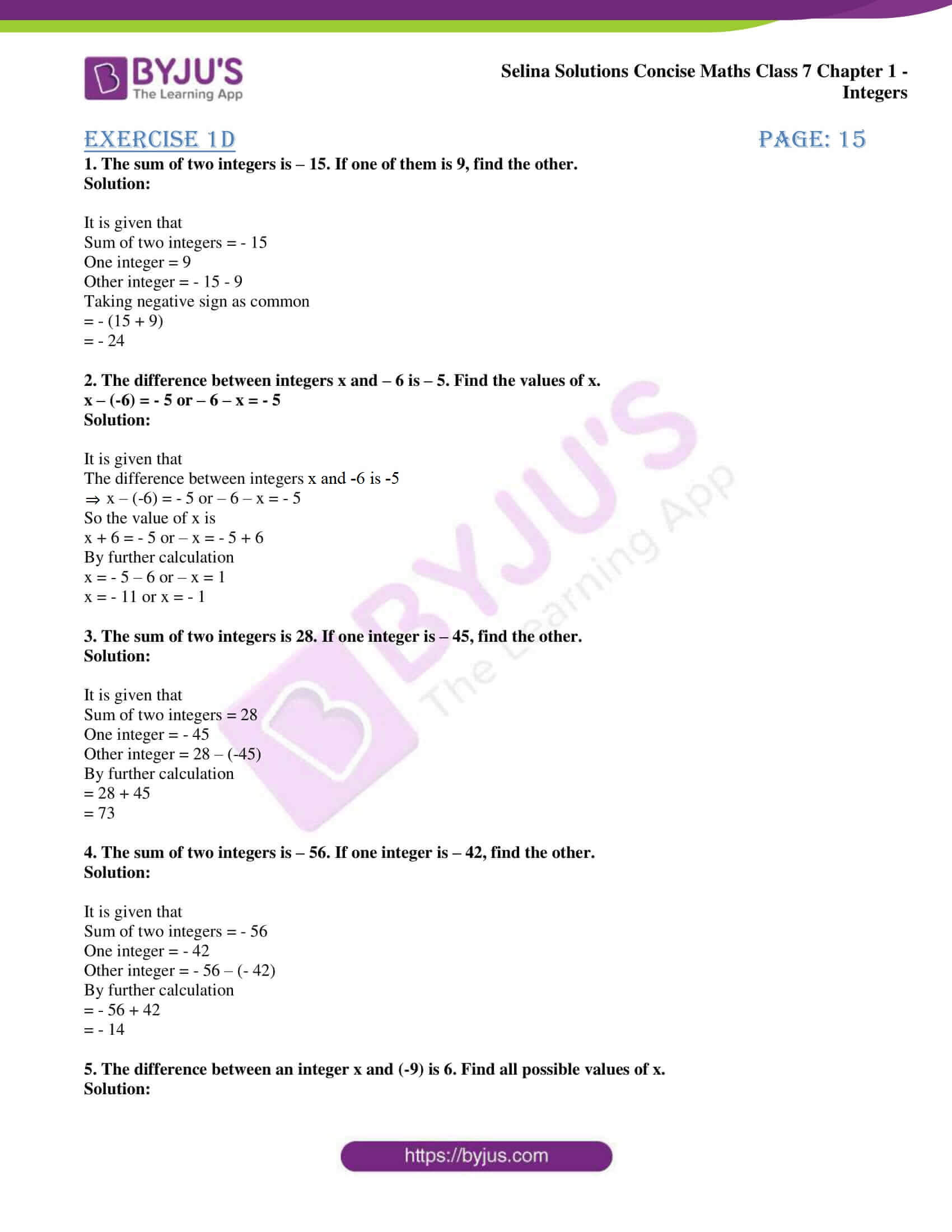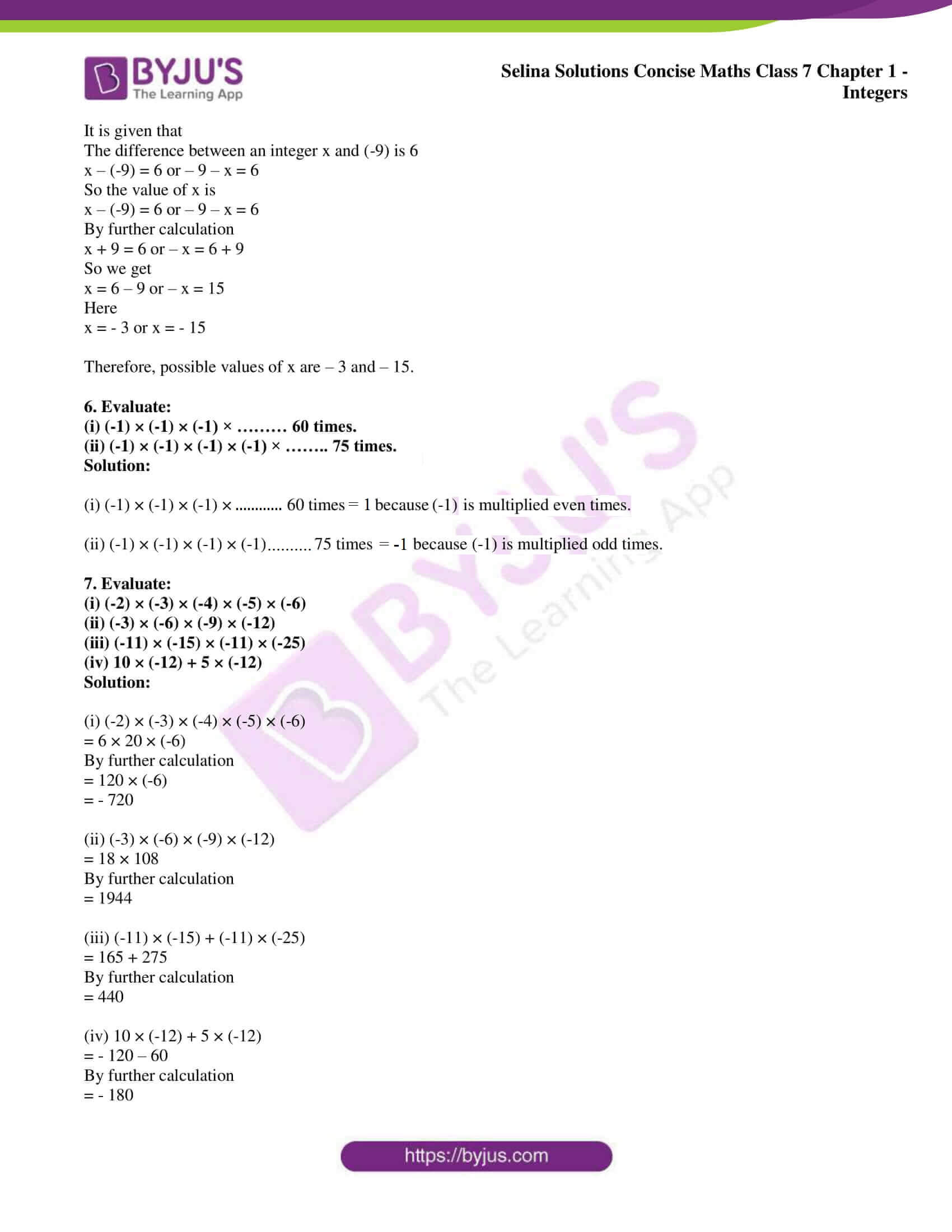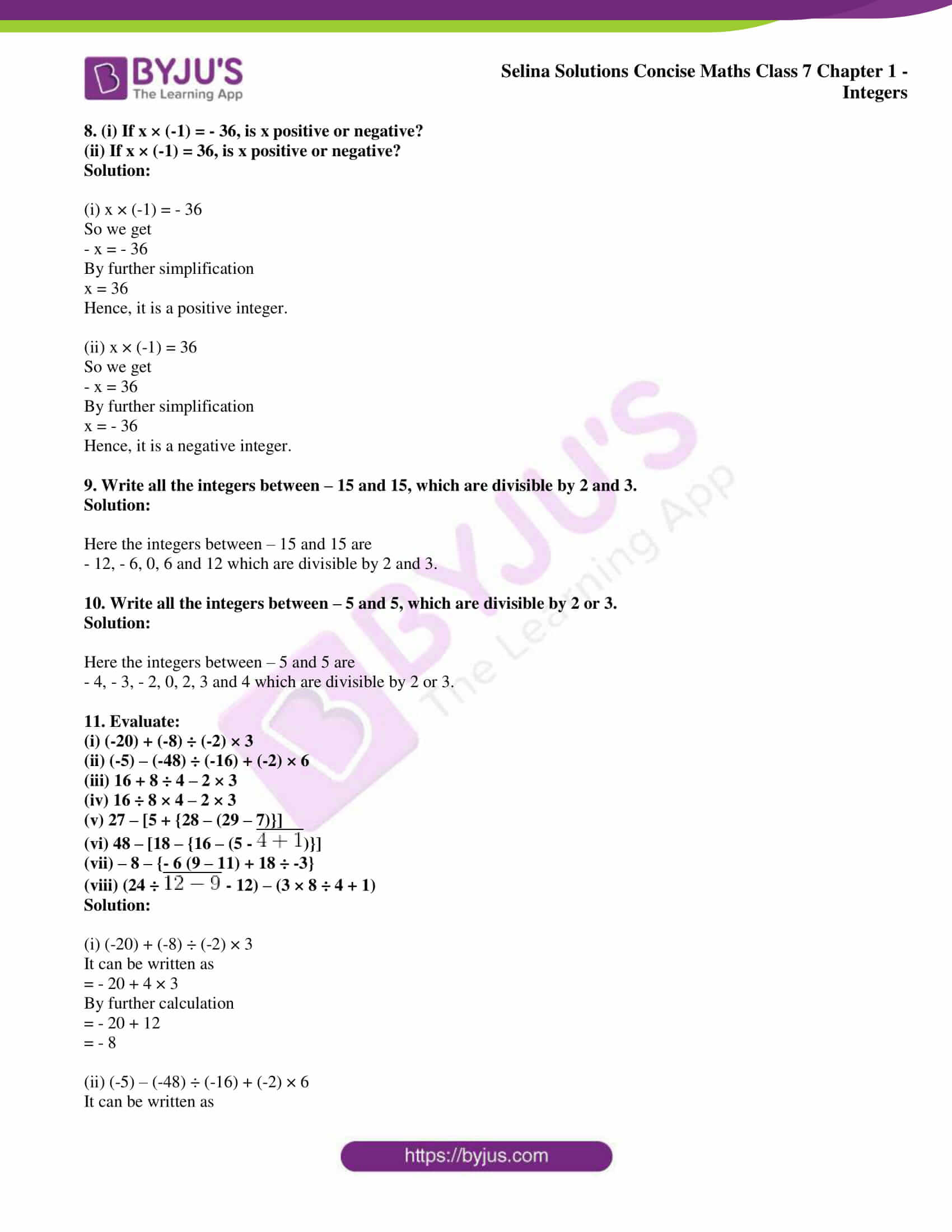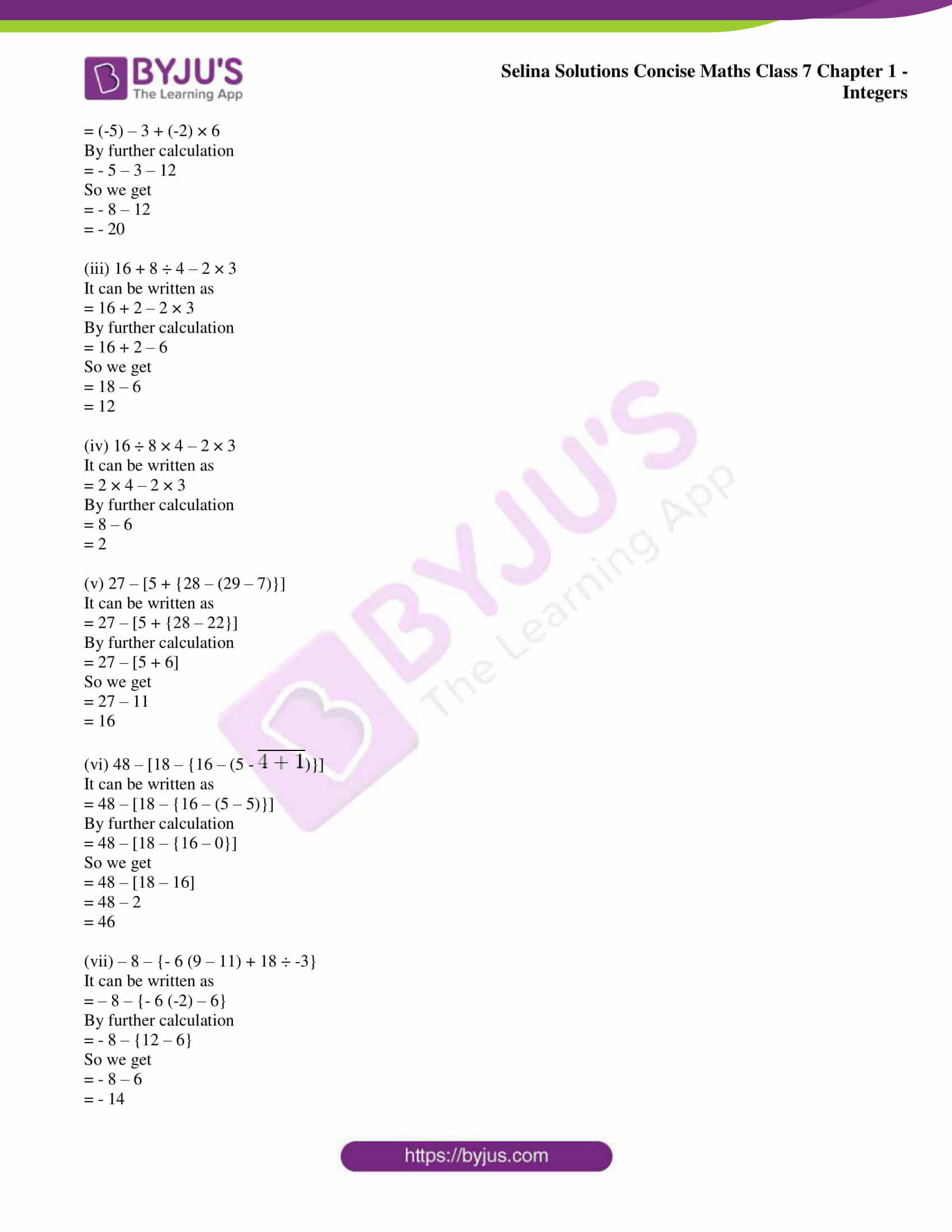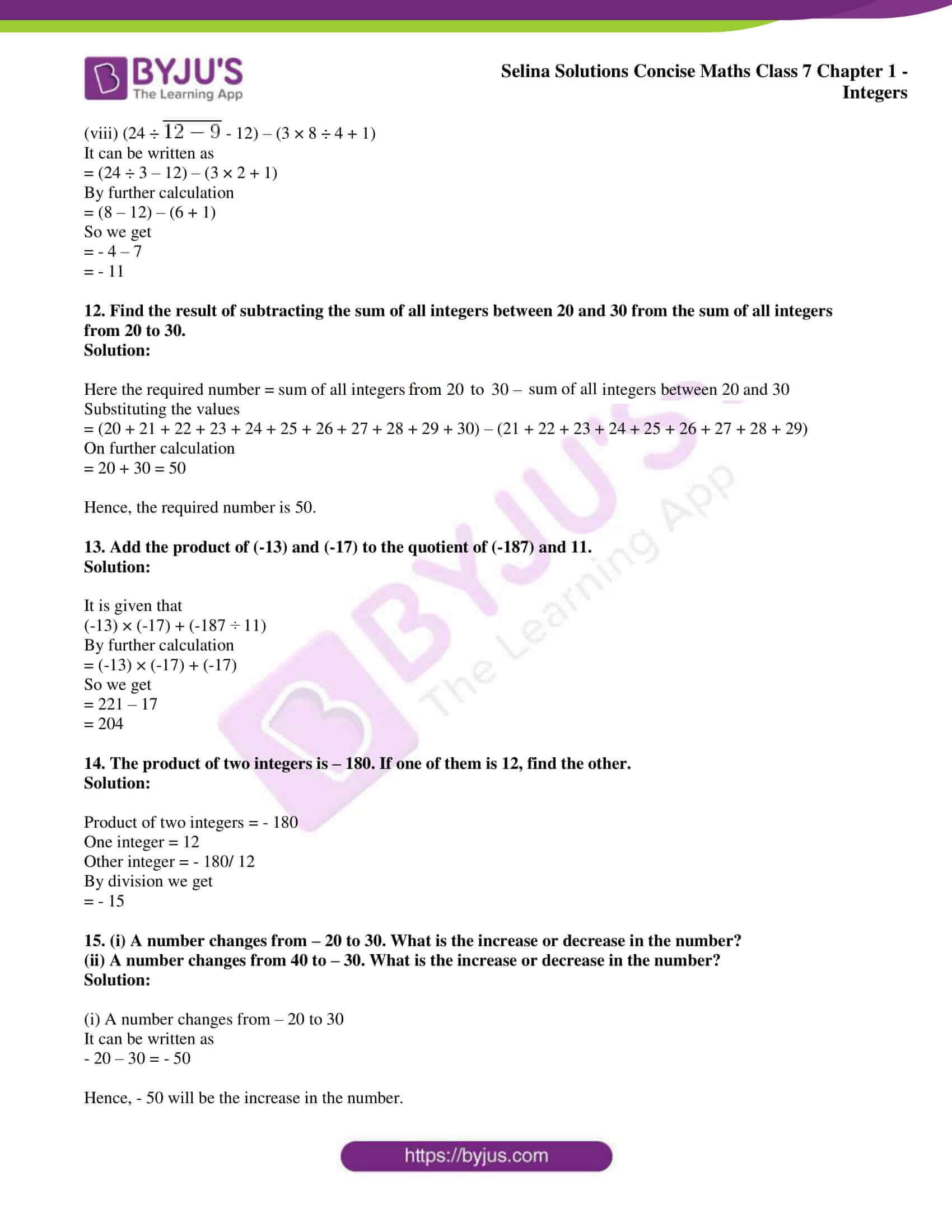### Access other exercises of Selina Solutions Concise Maths Class 7 Chapter 1: Integers

Exercise 1A Solutions

Exercise 1B Solutions

Exercise 1C Solutions

### Access Selina Solutions Concise Maths Class 7 Chapter 1: Integers Exercise 1D

1. The sum of two integers is – 15. If one of them is 9, find the other.

Solution:

It is given that

Sum of two integers = – 15

One integer = 9

Other integer = – 15 – 9

Taking negative sign as common

= – (15 + 9)

= – 24

2. The difference between integers x and – 6 is – 5. Find the values of x.

x – (-6) = – 5 or – 6 – x = – 5

Solution:

It is given that

The difference between integers x and -6 is -5

⇒ x – (-6) = – 5 or – 6 – x = – 5

So the value of x is

x + 6 = – 5 or – x = – 5 + 6

By further calculation

x = – 5 – 6 or – x = 1

x = – 11 or x = – 1

3. The sum of two integers is 28. If one integer is – 45, find the other.

Solution:

It is given that

Sum of two integers = 28

One integer = – 45

Other integer = 28 – (-45)

By further calculation

= 28 + 45

= 73

4. The sum of two integers is – 56. If one integer is – 42, find the other.

Solution:

It is given that

Sum of two integers = – 56

One integer = – 42

Other integer = – 56 – (- 42)

By further calculation

= – 56 + 42

= – 14

5. The difference between an integer x and (-9) is 6. Find all possible values of x.

Solution:

It is given that

The difference between an integer x and (-9) is 6

x – (-9) = 6 or – 9 – x = 6

So the value of x is

x – (-9) = 6 or – 9 – x = 6

By further calculation

x + 9 = 6 or – x = 6 + 9

So we get

x = 6 – 9 or – x = 15

Here

x = – 3 or x = – 15

Therefore, possible values of x are – 3 and – 15.

6. Evaluate:

(i) (-1) × (-1) × (-1) × ……… 60 times.

(ii) (-1) × (-1) × (-1) × (-1) × …….. 75 times.

Solution:

(i) (-1) × (-1) × (-1) × ……. 60 times = 1 because (-1) is multiplied even number of times.

(ii) (-1) × (-1) × (-1) × (-1) × …….. 75 times = -1 because (-1) is multiplied odd number of times.

7. Evaluate:

(i) (-2) × (-3) × (-4) × (-5) × (-6)

(ii) (-3) × (-6) × (-9) × (-12)

(iii) (-11) × (-15) × (-11) × (-25)

(iv) 10 × (-12) + 5 × (-12)

Solution:

(i) (-2) × (-3) × (-4) × (-5) × (-6)

= 6 × 20 × (-6)

By further calculation

= 120 × (-6)

= – 720

(ii) (-3) × (-6) × (-9) × (-12)

= 18 × 108

By further calculation

= 1944

(iii) (-11) × (-15) + (-11) × (-25)

= 165 + 275

By further calculation

= 440

(iv) 10 × (-12) + 5 × (-12)

= – 120 – 60

By further calculation

= – 180

8. (i) If x × (-1) = – 36, is x positive or negative?

(ii) If x × (-1) = 36, is x positive or negative?

Solution:

(i) x × (-1) = – 36

So we get

– x = – 36

By further simplification

x = 36

Hence, it is a positive integer.

(ii) x × (-1) = 36

So we get

– x = 36

By further simplification

x = – 36

Hence, it is a negative integer.

9. Write all the integers between – 15 and 15, which are divisible by 2 and 3.

Solution:

Here the integers between – 15 and 15 are

– 12, – 6, 0, 6 and 12 which are divisible by 2 and 3.

10. Write all the integers between – 5 and 5, which are divisible by 2 or 3.

Solution:

Here the integers between – 5 and 5 are

– 4, – 3, – 2, 0, 2, 3 and 4 which are divisible by 2 or 3.

11. Evaluate:

(i) (-20) + (-8) ÷ (-2) × 3

(ii) (-5) – (-48) ÷ (-16) + (-2) × 6

(iii) 16 + 8 ÷ 4 – 2 × 3

(iv) 16 ÷ 8 × 4 – 2 × 3

(v) 27 – [5 + {28 – (29 – 7)}]

(vi) 48 – [18 – {16 – (5 –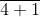)}]

(vii) – 8 – {- 6 (9 – 11) + 18 ÷ -3}

(viii) (24 ÷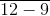– 12) – (3 × 8 ÷ 4 + 1)

Solution:

(i) (-20) + (-8) ÷ (-2) × 3

It can be written as

= – 20 + 4 × 3

By further calculation

= – 20 + 12

= – 8

(ii) (-5) – (-48) ÷ (-16) + (-2) × 6

It can be written as

= (-5) – 3 + (-2) × 6

By further calculation

= – 5 – 3 – 12

So we get

= – 8 – 12

= – 20

(iii) 16 + 8 ÷ 4 – 2 × 3

It can be written as

= 16 + 2 – 2 × 3

By further calculation

= 16 + 2 – 6

So we get

= 18 – 6

= 12

(iv) 16 ÷ 8 × 4 – 2 × 3

It can be written as

= 2 × 4 – 2 × 3

By further calculation

= 8 – 6

= 2

(v) 27 – [5 + {28 – (29 – 7)}]

It can be written as

= 27 – [5 + {28 – 22}]

By further calculation

= 27 – [5 + 6]

So we get

= 27 – 11

= 16

(vi) 48 – [18 – {16 – (5 –)}]

It can be written as

= 48 – [18 – {16 – (5 – 5)}]

By further calculation

= 48 – [18 – {16 – 0}]

So we get

= 48 – [18 – 16]

= 48 – 2

= 46

(vii) – 8 – {- 6 (9 – 11) + 18 ÷ -3}

It can be written as

= – 8 – {- 6 (-2) – 6}

By further calculation

= – 8 – {12 – 6}

So we get

= – 8 – 6

= – 14

(viii) (24 ÷– 12) – (3 × 8 ÷ 4 + 1)

It can be written as

= (24 ÷ 3 – 12) – (3 × 2 + 1)

By further calculation

= (8 – 12) – (6 + 1)

So we get

= – 4 – 7

= – 11

12. Find the result of subtracting the sum of all integers between 20 and 30 from the sum of all integers from 20 to 30.

Solution:

Here the required number = sum of all integers from 20 to 30 – sum of all integers between 20 and 30

Substituting the values

= (20 + 21 + 22 + 23 + 24 + 25 + 26 + 27 + 28 + 29 + 30) – (21 + 22 + 23 + 24 + 25 + 26 + 27 + 28 + 29)

On further calculation

= 20 + 30 = 50

Hence, the required number is 50.

13. Add the product of (-13) and (-17) to the quotient of (-187) and 11.

Solution:

It is given that

(-13) × (-17) + (-187 ÷ 11)

By further calculation

= (-13) × (-17) + (-17)

So we get

= 221 – 17

= 204

14. The product of two integers is – 180. If one of them is 12, find the other.

Solution:

Product of two integers = – 180

One integer = 12

Other integer = – 180/ 12

By division we get

= – 15

15. (i) A number changes from – 20 to 30. What is the increase or decrease in the number?

(ii) A number changes from 40 to – 30. What is the increase or decrease in the number?

Solution:

(i) A number changes from – 20 to 30

It can be written as

– 20 – 30 = – 50

Hence, – 50 will be the increase in the number.

(ii) A number changes from 40 to – 30

It can be written as

40 – (-30) = 40 + 30 = 70

Hence, 70 will be the decrease in the number.